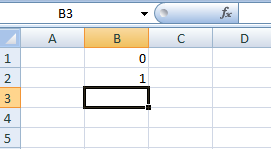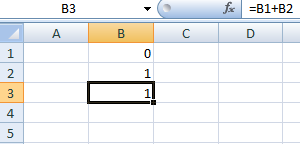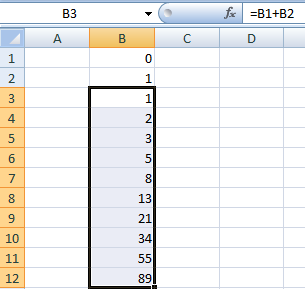### Fibonacci Sequence in MS Excel

In this section, you will be learning how to generate Fibonacci Sequence using Excel.
we all know, the Fibonacci sequence is of the form:  f(n) = f(n-1) + f(n-2)
Fibonacci sequence is characterized by the fact that every number after the first two is the sum of the two preceding ones.  You can use the equation above to obtain the rest of the Fibonacci numbers. It's easy to create Fibonacci sequences in Excel.
Let's learn with example
1. The first two numbers in the Fibonacci sequence are 0 and 1.2. Now , every number after the first two can be found by adding up the two previous numbers.3. Click on the lower right corner of cell B3 and drag it down.Result will be as above.
If you want more number in series, continue to drag it further & you will get the Fibonacci sequence in excel.